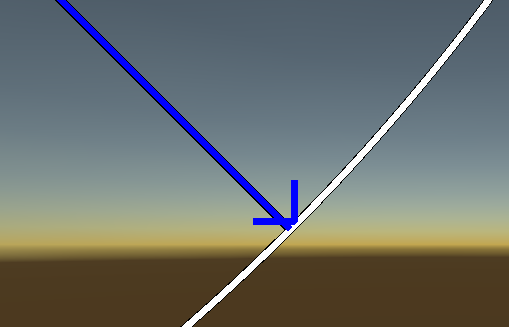# I have questions about ui linerender and linerender.I’d like to draw a directional arrow with ui linerender. An angle is inputted and rotated using recttransform.rotation, but the line does not continue to 0,0 coordinates.
Also, even though you specify the line as a parent and the child as an arrow shape, the more you zoom in, the less you draw it properly. Is there any other solution?

There’s some part of code.

``````UILineRenderer line;
public UILineRenderer line2;
public float width;
float standardWidth;
[Range(0, 360)]
public float angle;
float lineLength;
float fixedScale;
RectLine rectline;
float alpha;

float arrowAngleX, arrowAngleY;
private void Awake()
{
rectline = GetComponentInChildren<RectLine>();
line = GetComponent<UILineRenderer>();
}
public void ShootingLineMake(float radius, float distance, float scale = 1)
{
alpha = 1.0f;
fixedScale = scale;
arrowAngleX = Mathf.Cos(45 * Mathf.Deg2Rad) * lineLength * 0.05f;
arrowAngleY = Mathf.Sin(45 * Mathf.Deg2Rad) * lineLength * 0.05f;

if (lineLength * Mathf.Cos(0) != 255.0f)
{
alpha = 2.0f;
}
line.Points = Vector2.zero;
line.Points = lineLength * Mathf.Cos(0) * Vector2.up;
line2.Points = line.Points + alpha * fixedScale * new Vector2(-arrowAngleX, -arrowAngleY) * 2.0f;
line2.Points = line.Points;
line2.Points = line.Points + alpha * fixedScale * new Vector2(arrowAngleX, -arrowAngleY) * 2.0f;
GetComponent<RectTransform>().rotation = Quaternion.Euler(0, 0, (-angle));
}
``````

I solved it.
The coordinates of the vertex were drawn at an angle of 45 degrees.